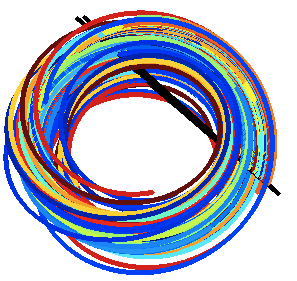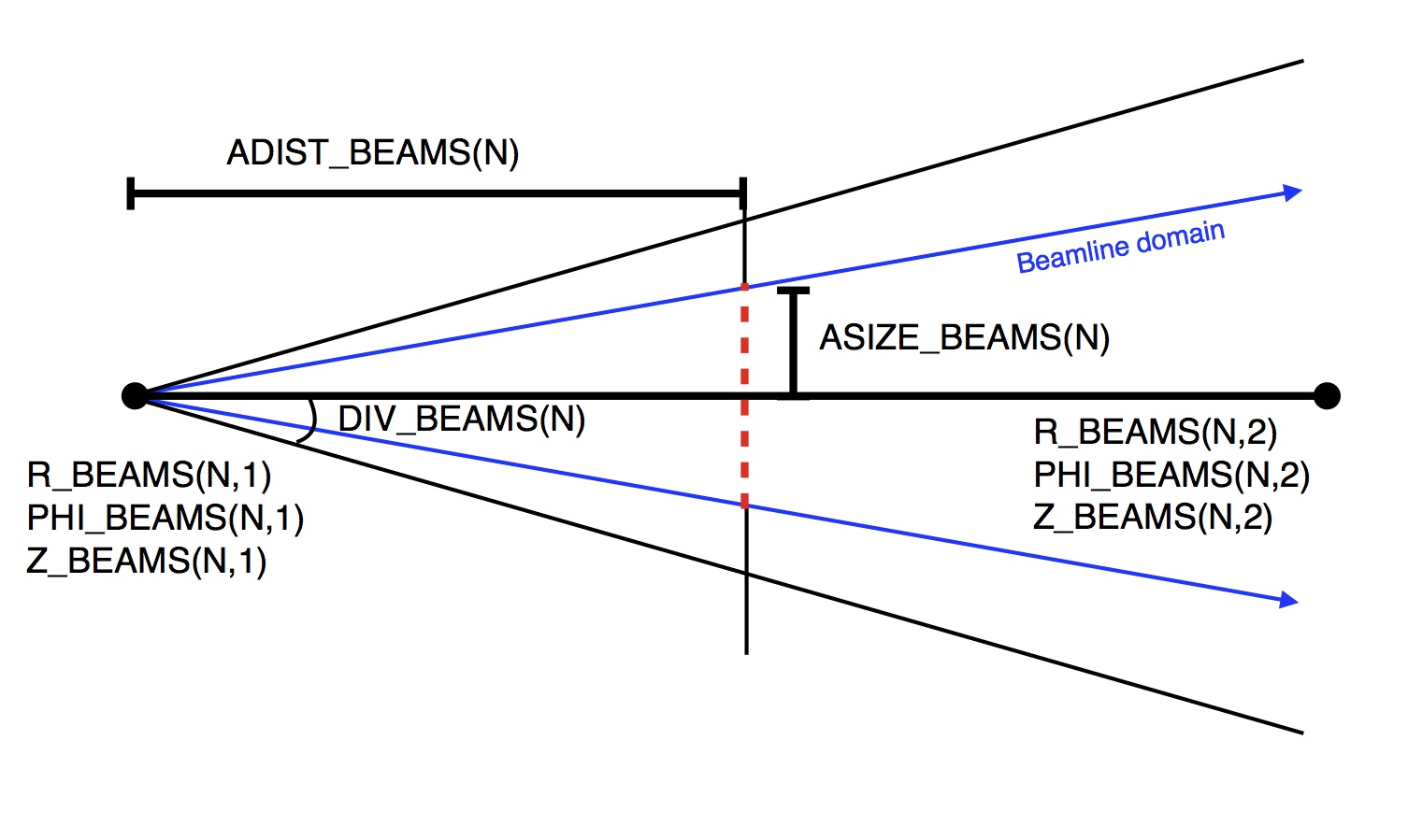# STELLOPT

State-of-the-art stellarator optimization code

# BEAMS3D

The BEAMS3D (Matthew McMillan and Samuel A Lazerson 2014 Plasma Phys. Control. Fusion 56 095019) code is a guiding center particle code capable of following both user defined ensembles of particles and modeling neutral beam injection in parallel. The magnetic field is represented by a three dimensional splines over a cylindrical grid. It is currently interfaced to the MAKEGRID coils file, MAKEGRID output file, and VMEC equilibria. Ionization and recombination models are provided by ADAS.

### TheoryThe BEAMS3D code follows the guiding center orbit equations on a cylindrical grid $$\frac{d\vec{R}}{dt}=\frac{\hat{b}}{qB}\left(\mu\nabla B +\frac{mv_{ll}^2}{2B}\left(\hat{b}\cdot\nabla\right)\vec{B}\right)+v_{ll}\hat{b}$$, $$\frac{dv_{ll}}{dt}=-\frac{\mu}{m}\hat{b}\cdot\left(\nabla B\right)$$. These ODE's can be solved via a NAG routine, LSODE, or Runge-Kutta algorithm. The magnetic field is splined over the cylindrical grid (R,phi,Z). The initial position and velocity of the particles can either be specified or modeled using a neutral beam model. The neutral beam model relies on ADAS for ionization and recombination physics. $$\mu = \frac{mv_\perp^2}{2B}$$

### Compilation

BEAMS3D is distributed as part of the STELLOPT package of codes through Git.

### Input Data Format

The BEAMS3D code is controlled through command line inputs and an input namelist which should be placed in the input.ext file. While the entire VMEC input name is not required some parts will be read. The name lists should look like:

&INDATA
! VMEC input namelist (only need coil currents for usual runs)
EXTCUR(1) = 10000.00
EXTCUR(2) = 10000.00
EXTCUR(3) = 12000.00
EXTCUR(4) = 12000.00
EXTCUR(5) = 6000.00
! VMEC Axis info (for putting a current on axis -axis option)
!     This information is utilized if you want to place the net toroidal current
!     on a magnetic axis.  Useful for doing vacuum tokamak equilibria
CURTOR = 5000.0
NFP = 5
NTOR = 6
RAXIS = 3.6  0.1 0.001
ZAXIS = 0.0  0.1 0.001
/
&BEAMS3D_INPUT
NR = 201                          ! Number of radial gridpoints, overridden if using mgrid
NPHI = 36                         ! Number of toroidal gridpoints, overridden if using mgrid
NZ = 201                          ! Number of vertical gridpoints, overridden if using mgrid
RMIN = 2.5                        ! Minimum extent of radial grid, overridden if using mgrid
RMAX = 5.0                        ! Maximum extent of radial grid, overridden if using mgrid
ZMIN = -1.5                       ! Minimum extent of vertical grid, overridden if using mgrid
ZMAX = 1.5                        ! Maximum extent of radial grid, overridden if using mgrid
PHIMIN = 0.0                      ! Minimum extent of toroidal grid, overridden if using mgrid
PHIMAX = 0.628                    ! Maximum extent of toroidal grid, overridden if using mgrid
R_START_IN =  3.6  3.7  3.8       ! Radial starting locations of particles [m]
Z_START_IN =  0.0  0.0  0.0       ! Vertical starting locations of particles [m]
PHI_START_IN =  0.0  0.0  0.0     ! Toroidal starting locations of particles (radians)
VLL_START_IN =  1.0E6 1.0E6 1.0E6 ! Initial parallel velocity of particles [m/s]
MU_START_IN =   3*1.0E-15         ! Particle magnetic moment [J/T] (0.5*m*v^2/B)
CHARGE_IN   =   3*1.60217733E-19  ! Particle charge [C]
MASS_IN     =   3*1.6726231E-27   ! Particle mass [kg]
ZATOM_IN    =   3*1.0             ! Particle charge number
T_END_IN    =   3*0.001           ! How long to follow particles [s]
NPOINC = 500                      ! Number of toroidal points per-period to output the field line trajectory
INT_TYPE = 'NAG'                  ! Particle trajectory integration method (NAG, RKH68, LSODE)
FOLLOW_TOL = 1.0E-12              ! Trajectory following tolerance
VC_ADAPT_TOL = 1.0E-7             ! Virtual casing tolerance (if using plasma field from equilibria)
! The following is used if modeling beam deposition as well
NPARTICLES_START = 500            ! Number of particles per beam line
ASIZE_BEAMS = 0.15                ! Aperature Size [m]
ADIST_BEAMS = 2.0                 ! Aperature Distance [m]
DIV_BEAMS = 0.1                   ! Beam divergence [rad] (small angle approximation used)
E_BEAMS   = 6.408E-15             ! Beam energy [J]
MASS_BEAMS = 1.6726231E-27        ! Beam particle mass [kg]
CHARGE_BEAMS = 1.60217733E-19     ! Beam particle charge [C]
ZATOM_BEAMS = 1.0                 ! Beam particle charge number
R_beams(1,1) = 1.673              ! Neutral beam radial position (Beam #1)
R_beams(1,2) = 1.400              ! Point defining beam line (Beam #1)
Z_beams(1,1) = 0.0                ! Neutral beam vertical position (Beam #1)
Z_beams(1,2) = 0.0                ! Point defining beam line (Beam #1)
PHI_BEAMS(1,1) = 0.0              ! Neutral beam toroidal poition (Beam #1)
PHI_BEAMS(1,2) = 1.047            ! Point defining beam line (Beam #1)
ADIST_BEAMS = 0.1                 ! Beam Appearture distance
TE_AUX_S  = 0.0 0.5 1.0           ! Electron Temperature Profile (radial knots)
TE_AUX_F  = 0.0 1.0 2.0           ! Electron Temperature Profile (values)
NE_AUX_S  = 0.0 0.5 1.0           ! Electron Density Profile (radial knots)
NE_AUX_F  = 0.0 1.0 2.0           ! Electron Density Profile (values)
TI_AUX_S  = 0.0 0.5 1.0           ! Ion Temperature Profile (radial knots)
TI_AUX_F  = 0.0 1.0 2.0           ! Ion Temperature Profile (values)
/
&END


It is important to note that neutral beam lines are defined by two points. The first index of the array is the beam number, the second defines the two points. The first point (X,1) is the origin on the beam. The second points (X,2) defines the beamline from that origin.### Execution

The BEASM3D code is controlled through a combination of command-line inputs and an input namelist. The input namelist must be in the equilibrium input file. That file must also contain the VMEC INDATA namelist (although only the EXTCUR array will be used). The BEASM3D code is run from the command line taking an equilibrium input file as a necessary argument. This input file must have the INDATA (for EXTCUR) and BEASM3D_INPUT namelists in it.

BEASM3D -vmec <VMEC FILE> -coil <COIL FILE> -mgrid <MGRID FILE> -vessel <VESSEL FILE> -vac -full -noverb -help

Argument Default Description
-vmec NONE VMEC input extension
-coil NONE Coils File
-mgrid NONE Makegrid style vacuum grid file
-vessel NONE First wall file
-beamlet NONE Beamlet deffintion HDF5 file.
-vac NONE Only compute the vacuum field
-beam_simple NONE Assume monoenergetic beams (normally 1% variance around injection energy)
-collisions NONE Force use of slowing down/scattering operator.
-depo NONE Calculate deposition only
-field NONE Outputs the B-Field on the cylindrical grid only.
-ascot4 NONE Creates input HDF5 file for ASCOT4 (BBNBI, no particles)
-ascot5 NONE Creates input HDF5 file for ASCOT5.
-hitonly NONE Only save vessel strike points.
-plasma NONE Only compute fields inside the plasma domain (places wall at LCFS)
-raw NONE Treats EXTCUR array as raw values (EXTCUR is a scale factor applied to what's in the coils file).
-w7x NONE Use W7-X beam shape model.
-noverb NONE Suppresses screen output
-help NONE Print help message.

In it's simplest invokation the code requires a VMEC input file.

>~/bin/xbeams3d -vmec ncsx_c09r00_free -mgrid mgrid_c09r00.nc -vac
BEAMS3D Version 1.00
----- Particle Initialization -----
S   = [  0.24490,  0.24490];   NS:        1
U   = [  0.00000,  5.96903];   NU:     20
V   = [  0.00000,  5.96903];   NV:     20
V_||= [*********,  2.00E+05];  NP:     10
Mu  = [  0.00000,  2.00E-15];  NP:     10
----- Profile Initialization -----
Ne  = [   0.00,   5.00] 10^19 [m^-3];  Nne:     50
Te  = [   0.00,   4.58] [keV];  Nte:     50
Ti  = [   0.00,   4.58] [keV];  Nti:     50
----- Input Parameters -----
R   = [  0.95223,  1.82142];  NR:    201
PHI = [ 0.00000, 2.09440];  NPHI:   60
Z   = [-0.66634, 0.66634];  NZ:    201
# of Particles to Start:   4000
----- Constructing Splines -----
R   = [  0.95223,  1.82142];  NR:    201
PHI = [ 0.00000, 2.09440];  NPHI:   60
Z   = [-0.66634, 0.66634];  NZ:    201
HERMITE FORM: 1
----- FOLLOWING PARTICLE TRAJECTORIES -----
Method: NAG
Particles: 4000
Steps:     320   Delta-t: 0.3125E-06
NPOINC:     320    dt_out: 0.1000E-06
Tol: 0.1000E-08  Type: M
----- WRITING DATA TO FILE -----
FILE: beams3d_ncsx_c09r00_free.h5
----- BEAMS3D DONE -----


### Output Data Format

The data from the run is output in two fashions. The first is a text file which contains counts of the number of particles lost from the simulation domain (hit a structure or the simulation domain). The second is and HDF5 file containing the simulation domain (magnetic fields on a grid) and the particle trajectories as a set of points. Each particle has it's trajectory saved NPOINC times.

### Visualization

The data from the HDF5 file may be easily plotted in many plotting packages. The R , PHI, Z, and V_PARALLEL coordinates of each particle are save NPOINC times along the trajectory. To aid in plotting the evolution of the distribution function the code outputs a textfile which bins by VLL the particles at each NPOINC time step.

Movie of Simulation

### Tutorials

NCSX Neutral Beam Injection Example